×

Search anything:

# Lomuto Partition Scheme

#### Algorithms Interview Problems on Array List of Mathematical AlgorithmsIn this article, we have explained the Lomuto partition scheme, which is used in the famous Quicksort algorithm. It is an algorithm to partition an array into two parts based on a given condition.

1. Background and Introduction
2. Algorithm
3. Quick sort using Lomuto Partition Scheme - Visualization
4. Working program
5. Comparison with Hoare Partition Scheme
6. Conclusion

# 1. Background and Introduction

Lomuto partition technique was made by Nico Lomuto and then eventually made its way into the spotlight when John Bentley included it in his book, Programming Pearls.

In the Lomuto partition scheme, usually the last element of the list is chosen as the pivot element.

Two pointers are also maintained for tracking and comparison purposes. A pointer i is maintained while another pointer j is scanning through the array, from its starting all the way to the end (up to the pivot element). The scan ensures that any element e₀ that is present from the starting point of the array to the (i-1)ᵗʰ index is lesser than the pivot element in terms of value and the elements present at any index ranging from i to j are equal to or bigger than the pivot element in terms of value.

Through this technique, the array will be sorted by the time we reach the end of the array.

# 2. Algorithm

The algorithm for the Lomuto partition scheme has been written below.

``````define lomutoPartition(A[], low, high) as:
put pivot = A[high]
put i = low-1
for j from low to (high-1) do:
if pivot >= A[j] then do:
Increment the value of i by 1
swap A[j] with A[i]
swap A[i+1] with the pivot element at A[high]
return the value of (i+1)
``````

# 3. Quick sort using Lomuto Partition Scheme - Visualization

• To demonstrate the working of the Lomuto Partition Scheme through quicksort, let us take an array:
A = [4, 2, 7, 3, 1, 9, 6, 0, 8]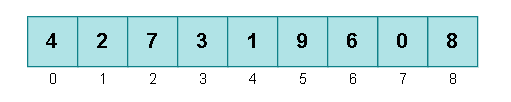• Choosing our pivot element as pivot = 8 and placing our initial pointers i and j (the arrow above the array is the for the i pointer whereas the arrow below the array is for the j pointer), we get: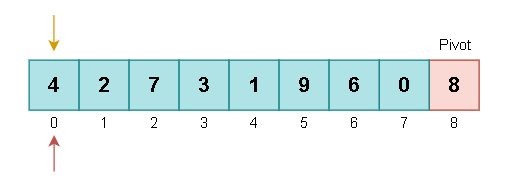• Now we have to simply follow the algorithm for the partition. The elements at the jth pointer will be compared to the pivot element continuously throughout the various iterations. Since pivot >= 4 (first element, where the pointers are pointing), we'll move the pointers ahead for the next comparison.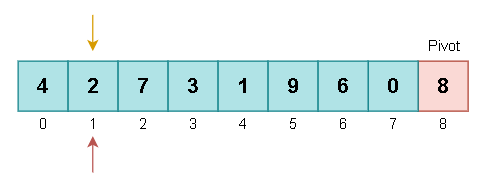• Once again, since pivot >= 2, we move ahead for the next comparison.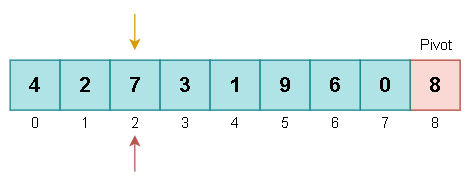• Here pivot >= 7 as well, so we keep on moving until we reach 9 element (5th index) of the array. Here, 9 >= pivot, and so we place one of our pointers at this index (5) until we reach another index that contains a value that is lesser than the pivot element or the pivot element itself.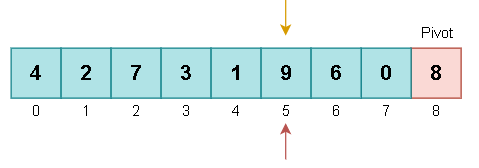• Moving ahead, we find that the element at the 6th index (6) is smaller than 9 (where we placed one of our pointers). A swapping operation is performed.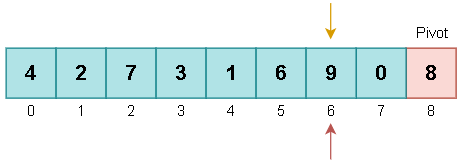• This process is repeated until we reach the pivot element, and so we will further go on and swap the values of 9 and 0, and then finally swap the values of 9 and the pivot element (8) itself. After the first pass, we get the following array: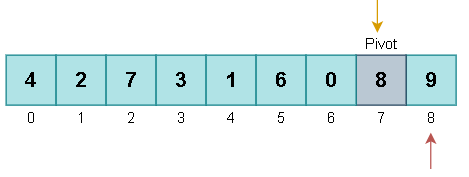If you notice carefully, all the elements present to the left of our current pivot element are smaller than the pivot element itself, whereas the elements present to the right of it are larger than the pivot element.

• We will now pick 0 as our pivot element from the array, pivot = 0.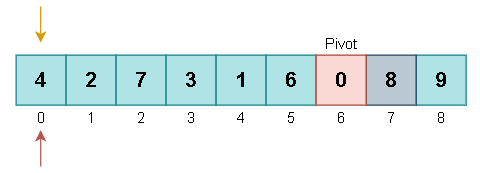• Once again, the same steps are going to be repeated until the pointers reach the pivot element. Since 4 >= pivot, we will place our ith pointer at the 0 index and then try to find another element along the way that is lesser than the pivot element. Seeing that no such element exists that is lesser than 0, we will have to swap the values of 0 and 4. After this operation has been performed, 4 will be chosen as our new pivot, pivot = 4.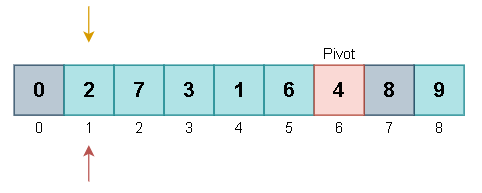• Following the same steps, this time the value of 7 is larger than our pivot element, and hence the following operations will be performed:
swap(7, 3) ==> swap(7, 1) ==> swap(7, 4)
After 7 is swapped with 4, 6 will be picked up as our next pivot element. Seeing that no remaining element is bigger than 6 in the range, we then make 1 as our pivot element, pivot = 1.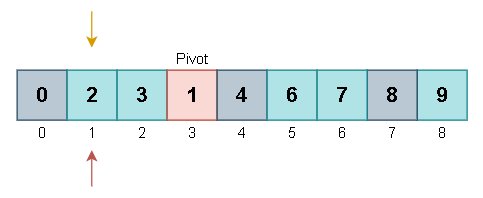• This time, the following operation will be performed:
swap(2, 1)
After this operation, 2 will be chosen as our pivot element, pivot = 2.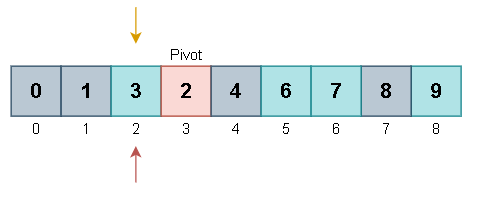• Finally, 2 will be swapped with 3 and we will have our final sorted array as follows: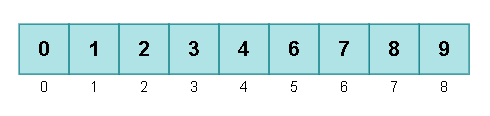# 4. Working program

``````def quicksort(A, low, high):
if low < high:
parti = lomutoPartition(A, low, high)
quicksort(A, low, parti-1)
quicksort(A, parti+1, high)

def lomutoPartition(A, low, high):
pivot = A[high]
i = low-1
for j in range(low, high):
if pivot >= A[j]:
i += 1
A[i], A[j] = A[j], A[i]
A[i+1], A[high] = A[high], A[i+1]
return i+1

if __name__ == "__main__":
A = [4, 2, 7, 3, 1, 9, 6, 0, 8]
low, high = 0, len(A)-1
print("BEFORE SORTING:", A)
quicksort(A, low, high)
print("AFTER SORTING:", A)
``````

The output generated is as follows:

``````BEFORE SORTING: [4, 2, 7, 3, 1, 9, 6, 0, 8]
AFTER SORTING: [0, 1, 2, 3, 4, 6, 7, 8, 9]
``````

# 5. Comparison with Hoare Partition Scheme

Brief overview of the Hoare Partition Scheme: Similar to the Lomuto partition scheme, the Hoare partition scheme also makes use of two pointers to partition the array. The pointers are placed at either ends of the array, i.e., one at the start and one at the end, and then they start moving towards each other while performing the comparisons.

The pivot element is usually taken to be the first element of the array, however, it is not restricted to that and other elements can be chosen as the pivot element as well.

The basic idea is that the pointers will keep on iterating through the elements until they come across a pair of elements where one element is greater than the pivot element whereas the other element is smaller than the pivot element and they are present in the wrong order relative to each other.

If such a pair is encountered, then a simple swap operation is performed and the elements of this pair are swapped with each other. These steps are repeated until the two pointers come across each other. When that happens, the algorithm can stop and then return the final index.

Hoare Partition Lomuto Partition
1 Makes use of two pointers for partitioning Also makes use of two pointers for partitioning
2 The first element of the array is
usually chosen as the pivot element,
although there is no restriction
The last element of the array is
usually chosen as the pivot element
although it can be random as well
3 It is a linear algorithm It is also a linear algorithm
4 It is more comparatively more efficient
and faster because of fewer swap operations
on average
It is more comparatively less efficient
and slower because of more swap operations
on average
5 It causes Quicksort to downgrade to `O(n²)`
if the array is already almost
or completely sorted
It also causes Quicksort to downgrade to `O(n²)`
if the array is already almost
or completely sorted
6 It is slightly complex to understand and implement It is comparatively easier to understand and implement

# 6. Conclusion

Lomuto partition scheme is very simple and easy to understand and implement. However, there are better alternatives present that can be used for the same purpose.

In Lomuto partition, the index variable j goes through the whole array and if the element A[j] is smaller than the pivot element p, a swap operation is performed. Among the elements 1,...,n, exactly (p-1) of them are smaller than the pivot element, and so we have total of (p-1) swap operations if pivot = p.

Hence, the average of all the pivots will result in the overall expected swaps. Each value from 1,...,n has the same likeliness of becoming the pivot element (with a probability of 1/n). We get: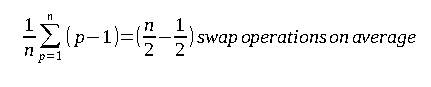where n = length of the array

The worst case complexity occurs when the array is already in order or almost sorted, the time complexity of this algorithm becomes `O(n²)`.

The Lomuto partition scheme can be used for various purposes including sorting the array, moving all even or odd elements to the front of the array, etc. The if conditions simply have to be modified a little to satisfy the required property or constraint, but the rest of the method remains the same and can be used for many purposes.

With this article at OpenGenus, you must have a strong idea of Lomuto Partition Scheme.#### Vansh Pratap Singh

Tough times don't last, tough people do. =)

Lomuto Partition Scheme